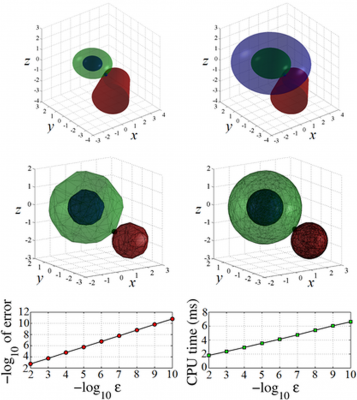## AbstractThis paper presents an efficient algorithm for computing a distance measure between two compact convex sets Q and A, defined as the minimum scale factor such that the scaled Q is not disjoint from A. An important application of this algorithm in robotics is the computation of the minimum distance between two objects, which can be performed by taking A as the Minkowski difference of the objects and Q as a set containing the origin in its interior. In this generalized definition, the traditional Euclidean distance is a special case where Q is the unit ball. While this distance measure was proposed almost a decade ago, there has been no efficient algorithm to compute it in general cases. Our algorithm fills this void and we demonstrate its superior efficiency compared to approaches based on general-purpose optimization.